# Maharashtra Board Class 10 Maths Solutions Chapter 1 Similarity Problem Set 1

## Maharashtra State Board Class 10 Maths Solutions Chapter 1 Similarity Problem Set 1

Question 1.
Select the appropriate alternative.
i. In ∆ABC and ∆PQR, in a one to one correspondence $$\frac { AB }{ QR }$$ = $$\frac { BC }{ PR }$$ = $$\frac { CA }{ PQ }$$, then(A) ∆PQR – ∆ABC
(B) ∆PQR – ∆CAB
(C) ∆CBA – ∆PQR
(D) ∆BCA – ∆PQR
(B)

ii. If in ∆DEF and ∆PQR, ∠D ≅ ∠Q, ∠R ≅ ∠E, then which of the following statements is false?(A) $$\frac { EF }{ PR }$$ = $$\frac { DF }{ PQ }$$
(B) $$\frac { DE }{ PQ }$$ = $$\frac { EF }{ RP }$$
(C) $$\frac { DE }{ QR }$$ = $$\frac { DF }{ PQ }$$
(D) $$\frac { EF }{ RP }$$ = $$\frac { DE }{ QR }$$
∆DEF ~ ∆QRP … [AA test of similarity]
∴ $$\frac { DE }{ QR }$$ = $$\frac { EF }{ RP }$$ = $$\frac { DF }{ PQ }$$ …[Corresponding sides of similar triangles]
(B)

iii. In ∆ABC and ∆DEF, ∠B = ∠E, ∠F = ∠C and AB = 3 DE, then which of the statements regarding the two triangles is true?(A) The triangles are not congruent and not similar.
(B) The triangles are similar but not congruent.
(C) The triangles are congruent and similar.
(D) None of the statements above is true.
(B)

iv. ∆ABC and ∆DEF are equilateral triangles, A(∆ABC) : A(∆DEF) = 1 : 2. If AB = 4, then what is length of DE?(A) 2√2
(B) 4
(C) 8
(D) 4√2
Refer Q. 6 Practice Set 1.4
(D)

v. In the adjoining figure, seg XY || seg BC, then which of the following statements is true?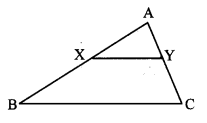(A) $$\frac { AB }{ AC }$$ = $$\frac { AX }{ AY }$$
(B) $$\frac { AX }{ XB }$$ = $$\frac { AY }{ AC }$$
(C) $$\frac { AX }{ YC }$$ = $$\frac { AY }{ XB }$$
(D) $$\frac { AB }{ YC }$$ = $$\frac { AC }{ XB }$$
∆ABC ~ ∆AXY … [AA test of similarity]
∴ $$\frac { AB }{ AX }$$ = $$\frac { AC }{ AY }$$ …[Corresponding sides of similar triangles]
∴ $$\frac { AB }{ AC }$$ = $$\frac { AX }{ AY }$$ …[Altemendo]
(A)

Question 2.
In ∆ABC, B-D-C and BD = 7, BC = 20, then find following ratios.Solution:Draw AE ⊥ BC, B – E – C.
BC = BD + DC [B – D – C]
∴ 20 = 7 + DC
∴ DC = 20 – 7 = 13

i. ∆ABD and ∆ADC have same height AE.
$$\frac{\mathrm{A}(\Delta \mathrm{ABD})}{\mathrm{A}(\Delta \mathrm{ADC})}=\frac{\mathrm{BD}}{\mathrm{DC}}$$ [Triangles having equal height]
∴ $$\frac{A(\Delta A B D)}{A(\Delta A D C)}=\frac{7}{13}$$

ii. ∆ABD and ∆ABC have same height AE.
$$\frac{\mathrm{A}(\Delta \mathrm{ABD})}{\mathrm{A}(\Delta \mathrm{ABC})}=\frac{\mathrm{BD}}{\mathrm{BC}}$$ [Triangles having equal height]
∴ $$\frac{A(\Delta A B D)}{A(\Delta A B C)}=\frac{7}{20}$$

iii. ∆ADC and ∆ABC have same height AE.
$$\frac{A(\Delta A D C)}{A(\Delta A B C)}=\frac{D C}{B C}$$ [Triangles having equal height]
∴ $$\frac{A(\Delta A D C)}{A(\Delta A B C)}=\frac{13}{20}$$

Question 3.
Ratio of areas of two triangles with equal heights is 2 : 3. If base of the smaller triangle is 6 cm, then what is the corresponding base of the bigger triangle?
Solution:
Let A1 and A2 be the areas of two triangles. Let b1 and b2 be their corresponding bases.
A1 : A2 = 2 : 3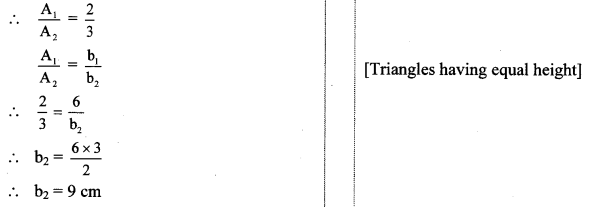∴ The corresponding base of the bigger triangle is 9 cm.

Question 4.
In the adjoining figure, ∠ABC = ∠DCB = 90°, AB = 6, DC = 8, then $$\frac{\mathbf{A}(\Delta \mathbf{A} \mathbf{B} \mathbf{C})}{\mathbf{A}(\mathbf{\Delta D C B})}=?$$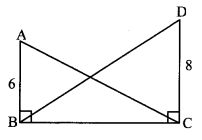Solution:
∆ABC and ∆DCB have same base BC.Question 5.
In the adjoining figure, PM = 10 cm, A(∆PQS) = 100 sq. cm,
A(∆QRS) = 110 sq. cm, then find NR.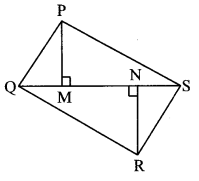Solution: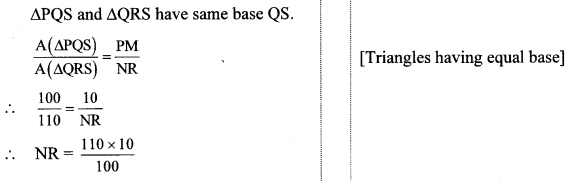∴ NR = 11 cm

Question 6.
∆MNT ~ ∆QRS. Length of altitude drawn from point T is 5 and length of altitude drawn from point S is 9. Find the ratio $$\frac{A(\Delta M N T)}{A(\Delta Q R S)}$$Solution:
∆MNT- ∆QRS [Given]
∴ ∠M ≅ ∠Q (i) [Corresponding angles of similar triangles]
In ∆MLT and ∆QPS,
∠M ≅ ∠Q [From (i)]
∠MLT ≅ ∠QPS [Each angle is of measure 90°]
∴ ∆MLT ~ ∆QPS [AA test of similarity]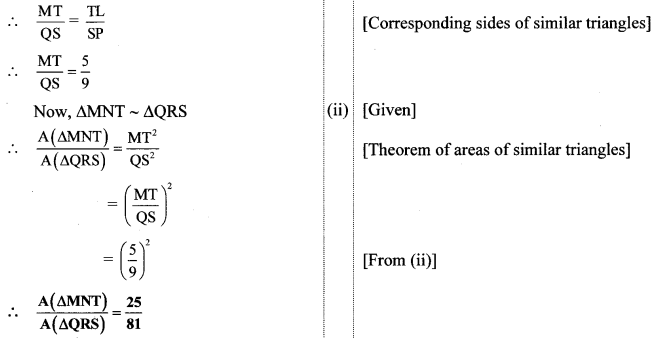Question 7.
In the adjoining figure, A – D – C and B – E – C. seg DE || side AB. If AD = 5, DC = 3, BC = 6.4, then find BE.Solution:
In ∆ABC,
seg DE || side AB [Given]
∴ $$\frac { DC }{ AD }$$ = $$\frac { EC }{ BE }$$ [Basic proportionality theorem]
∴ $$\frac { 3 }{ 4 }$$ = $$\frac { 6.4-x }{ x }$$
∴ 3x = 5 (6.4 – x)
∴ 3x = 32 – 5x
∴ 8x = 32
∴ x = $$\frac { 32 }{ 8 }$$ =4
∴ BE = 4 units

Question 8.
In the adjoining figure, seg PA, seg QB, seg RC and seg SD are perpendicular to line AD. AB = 60, BC = 70, CD = 80, PS = 280, then find PQ, QR and RS.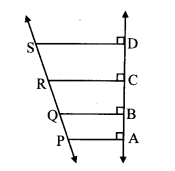Solution:
seg PA, seg QB, seg RC and seg SD are perpendicular to line AD. [Given]
∴ seg PA || seg QB || seg RC || seg SD (i) [Lines perpendicular to the same line are parallel to each other]
Let the value of PQ be x and that of QR be y.
PS = PQ + QS [P – Q – S]
∴ 280 – x + QS
∴ QS = 280 – x (ii)
Now, seg PA || seg QB || seg SD [From (i)]
∴ $$\frac { AB }{ BD }$$ = $$\frac { PQ }{ QS }$$ [Property of three parallel lines and their transversals]
∴$$\frac { AB }{ BC+CD }$$ = $$\frac { PQ }{ QS }$$ [B – C – D]
∴ $$\frac { 60 }{ 70+80 }$$ = $$\frac { x }{ 280-x }$$
∴ $$\frac { 60 }{ 150 }$$ = $$\frac { x }{ 280-x }$$
∴ $$\frac { 2 }{ 5 }$$ = $$\frac { x }{ 280-x }$$
∴ 5x = 2 (280 – x)
∴ 5x = 560 – 2x
∴ 7x = 560
∴ x = $$\frac { 560 }{ 7 }$$ = 80
∴ PQ = 80 units
QS = 280 – x [From (ii)]
= 280 – 80
= 200 units
But, QS = QR + RS [Q – R – S]
∴ 200 = y + RS
∴ RS = 200 – y (ii)
Now, seg QB || seg RC || seg SD [From (i)]
∴$$\frac { BC }{ CD }$$ = $$\frac { QR }{ RS }$$ [Property of three parallel lines and their transversals]
∴ $$\frac { 70 }{ 80 }$$ = $$\frac { y }{ 200-y }$$
∴ $$\frac { 7 }{ 8 }$$ = $$\frac { y }{ 200-y }$$
∴ 8y = 7(200 – y)
∴ 8y = 1400 – 7y
∴ 15y = 1400
∴ y = $$\frac { 1400 }{ 15 }$$ = $$\frac { 280 }{ 3 }$$
∴ QR = $$\frac { 280 }{ 3 }$$ units
RS = 200 – 7 [From (iii)]
= 200 – $$\frac { 280 }{ 3 }$$
= $$\frac{200 \times 3-280}{3}$$
= $$\frac { 600-280 }{ 3 }$$
∴ RS = $$\frac { 320 }{ 3 }$$ units

Question 9.
In ∆PQR, seg PM is a median. Angle bisectors of ∠PMQ and ∠PMR intersect side PQ and side PR in points X and Y respectively. Prove that XY || QR
Complete the proof by filling in the boxes.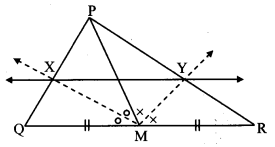Solution:
Proof:
In ∆PMQ, ray MX is bisector of ∠PMQ.Question 10.
In the adjoining figure, bisectors of ∠B and ∠C of ∆ABC intersect each other in point X. Line AX intersects side BC in point Y.
AB = 5, AC = 4, BC = 6, then find $$\frac { AX }{ XY }$$.Solution:
Let the value of BY be x.
BC = BY + YC [B – Y – C]
∴ 6 = x + YC
∴ YC = 6 – x
in ∆BAY, ray BX bisects ∠B. [Given]
∴ $$\frac { AB }{ BY }$$ = $$\frac { AX }{ XY }$$ (i) [Property of angle bisector of a triangle]
Also, in ∆CAY, ray CX bisects ∠C. [Given]Question 11.
In ꠸ABCD, seg AD || seg BC. Diagonal AC and diagonal BD intersect each other in point P. Then show that $$\frac { AP }{ PD }$$ = $$\frac { PC }{ BP }$$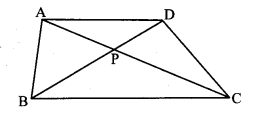Solution:
proof:
seg AD || seg BC and BD is their transversal. [Given]
∴ ∠DBC ≅ ∠BDA [Alternate angles]
∴ ∠PBC ≅ ∠PDA (i) [D – P – B]
In ∆PBC and ∆PDA,
∠PBC ≅ ∠PDA [From (i)]
∠BPC ≅ ∠DPA [Vertically opposite angles]
∴ ∆PBC ~ ∆PDA [AA test of similarity]
∴ $$\frac { BP }{ PD }$$ = $$\frac { PC }{ AP }$$ [Corresponding sides of similar triangles]
∴ $$\frac { AP }{ PD }$$ = $$\frac { PC }{ BP }$$ [By altemendo]

Question 12.
In the adjoining figure, XY || seg AC. If 2 AX = 3 BX and XY = 9, complete the activity to find the value of AC.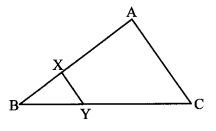Solution:
2 AX = 3 BX [Given]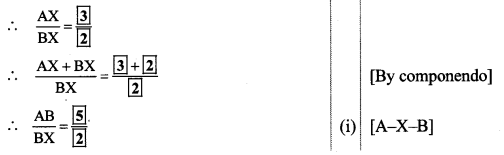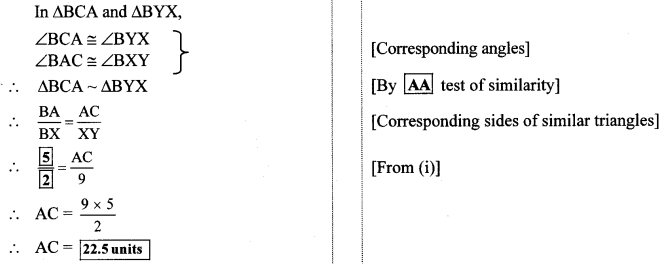Question 13.
In the adjoining figure, the vertices of square DEFG are on the sides of ∆ABC. If ∠A = 90°, then prove that DE2 = BD × EC.
(Hint: Show that ∆GBD is similar to ∆ CFE. Use GD = FE = DE.)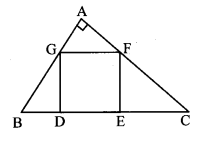Solution:
proof:
꠸DEFG is a square.
∴ DE = EF = GF = GD (i) [Sides of a square]
∠GDE = ∠DEF = 90° [Angles of a square]
∴ seg GD ⊥ side BC, seg FE ⊥ side BC (ii)
In ∆BAC and ∆BDG,
∠BAC ≅ ∠BDG [From (ii), each angle is of measure 90°]
∠ABC ≅ ∠DBG [Common angle]
∴ ∆BAC – ∆BDG (iii) [AA test of similarity]
In ∆BAC and ∆FEC,
∠BAC ≅ ∠FEC [From (ii), each angle is measure 90°]
∠ACB ≅ ∠ECF [Common angle]
∴ ∆BAC – ∆FEC (iv) [AA test of similarity]
∴ ∆BDG – ∆FEC [From (iii) and (iv)]
∴ $$\frac { BD }{ EF }$$ = $$\frac { GD }{ EC }$$ (v) [Corresponding sides of similar triangles]
∴ $$\frac { BD }{ DE }$$ = $$\frac { DE }{ EC }$$ [From (i) and (v)]
∴ DE2 = BD × EC